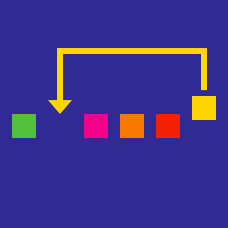Computer Science

# Counting Sort

Let $n$ be the size of an input array made up of positive integers and suppose that your algorithm is given $V_{max}$, the maximum value of all elements within the input array. Which of the following describes the correct space complexity of counting Sort in this case?

Suppose we have an array with $n$ elements and $k$ is the size of the largest key. When would it be a bad idea to use counting sort to sort this array?

Details and assumptions

$k = O(f(n))$ means $k$ is bounded by the function $f(n)$.

Assume we want to sort an array of ints. In which scenario is the use of counting sort the most sensible?

×